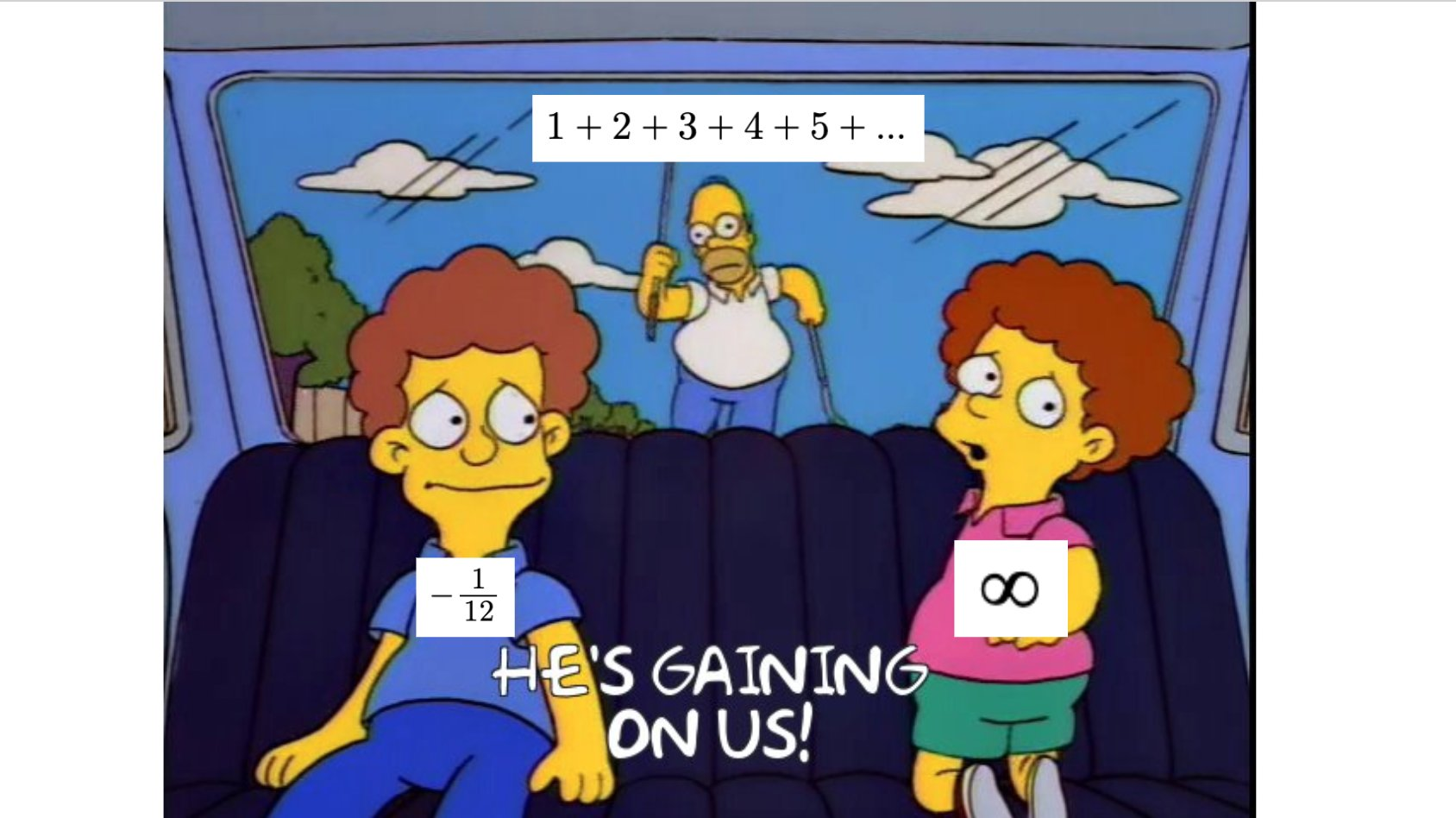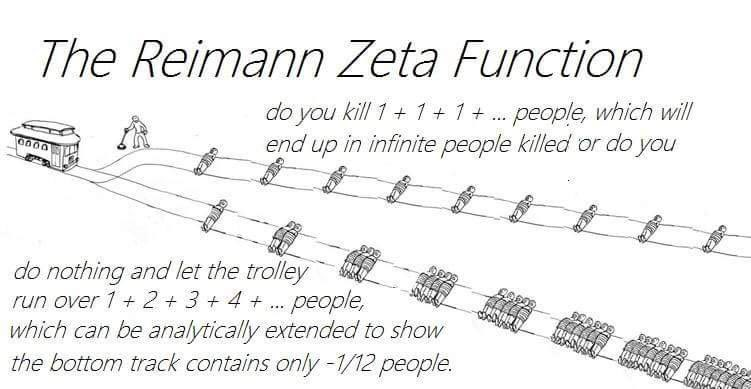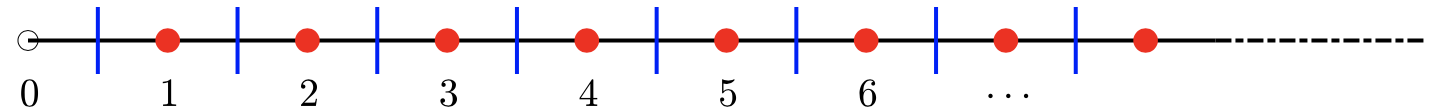Bobbie's Blog

Zeta Function = Sum - Integral

Recently I saw some memes about the peculiar “sum”These memes are inspired by the Riemann zeta function

in which directly substituting $s=-1$ gives the $-\tfrac{1}{12}$ “sum” above.

This $1+2+3+4+\dots = -1/12$ joke was first popularized by the 2014 numberphile video. The video went viral and caused a lot of confusions due to the lack of proper explanations.

Indeed, adding $1+2+3+4+\dots$ will blow up to infinity in the usual sense. The definition of $\zeta(s)$ by summing $1/n^s$ only makes sense for $s>1$; when $s<1$, the value $\zeta(s)$ is in fact obtained by analytic continuation. Later in 2018, Mathologer gave an accessible clarification which only requires a minimal background in calculus to understand.

A New Perspective on the Zeta Function

Here, I will provide another interesting perspective on the continuation of $\zeta(s)$, which I think is quite beautiful and deserves more attentions. This is stated in the title of this article:

zeta function $=$ sum $-$ integral.

Specifically,

This relationship holds for any $s$ in the complex plane $\mathbb{C}$, provided we define this expression appropriately.

The Proof

For simplicity, let’s start with $-1<\mathrm{Re}(s)<1$, which is a vertical strip in $\mathbb{C}$ where both the sum and the integral diverge. Then an appropriate zeta-sum-integral connection can be written as

To paint a clearer picture, let’s use some visual help and consider the above limit on the real line:Here, the red dots are the integer points $n = 1, 2, 3,\dots$, and the blue bars divide the real line into boxes of unit length $[\frac12, \frac32], [\frac32, \frac{5}{2}], [\frac{5}{2}, \frac{7}{2}], \dots$

Based on this picture, let’s first define some quantities of interest:

• Define the partial sum $S_N(s) := \sum_{n=1}^N\frac{1}{n^s}$ which is a sum on the first $N$ red dots in the picture above.
• Define the finite integral $I_N(s) := \int_{\frac12}^{N+\frac12}\frac{1}{x^s}\,dx$ which is an integral in the first $N$ boxes defined by the blue bars in the picture.
• Define the difference $Z_N(s) := S_N(s) - I_N(s)$, then

is a sum of $N$ items in the first $N$ boxes. The $n$th item $\Delta Z_n(s):=\frac{1}{n^s} - \int_{n-\frac12}^{n+\frac12}\frac1{x^s}\,dx$ is the difference between a term at the $n$th red dot and an integral over the containing blue box.

Next, we realize some nice properties of the sum $Z_N(s)$

• Looking at each term in the sum $Z_N(s)$

we find that for any fixed $n$, $\Delta Z_n(s)$ is an analytic function of $s\in\mathbb{C}$.

• By a comparison with $\sum_{n=1}^\infty\frac{1}{n^{s+2}}$, it is not hard to establish the convergence of $Z_\infty(s) = \sum_{n=1}^\infty\Delta Z_N(s)$ for all $\mathrm{Re}(s)>-1$. This indicates that $Z_\infty(s)$ is an analytic function for all $\mathrm{Re}(s)>-1$.

With these setup, let’s start our proof, which will be very straightforward.

• We first notice that

So $I_0(s)$ and $-J_0(s)$ are analytic continuations of each other to the respective half-planes. Consequently, the following two functions are also analytic continuations of each other

• Our goal is to show that

therefore we will be done once it is shown that $\zeta(s) = Z_\infty(s) + J_0(s)$ for $\mathrm{Re}(s)>1$. This can be shown simply:

Q.E.D.

Euler-Maclaurin Formula and the Zeta Function

The famous Euler-Maclarin formula is an important tool for approximating integrals on a uniform grid. Probably lesser-known is its connection to the zeta function.

The typical Euler-Maclaurin formula on the integer grid $n=0,1,2,\dots,N$ is

Using the relationship between the the Bernoulli numbers and the zeta function, we can rewrite this as

It is remarkable that we once again return to the “zeta = sum - integral” relation.

Let’s simplify this further. If we substitute $f(x) = x^k$ into the formula, a lot of the terms become zero and we get

Then we let $N\to\infty$ and think of the limiting integral as a Hadamard finite part integral (f.p.), this yields

This is exactly the “zeta = sum - integral” relation! So the Euler-Maclaurin formula is just a special case of this connection after all. (Alternatively, one can think of the finite part integral as two integrals $\int_0^1x^k\,dx$ and $\int_1^\infty x^k\,dx$, then use the analytic continuation idea, like for the $I_0$ and $J_0$ integrals in the above proof, to show that they add to $0$.)

We see that using appropriate notions, the relation

holds for all values of $s\in\mathbb{C}$.

I have written before about integrating singular functions using this zeta relation, but the Euler-Maclarin formula shows that such relation also works for regular integrals. So, and here is the punchline, an even more beautiful fact is that we can handle both singular and regular integrals categorically using just one zeta relation!1

1. For more general zeta function connections, see the recent book chapter by Avraham Sidi.